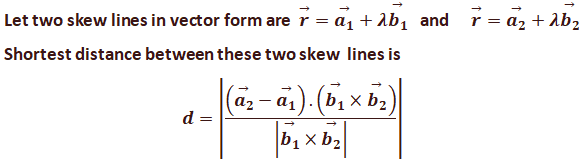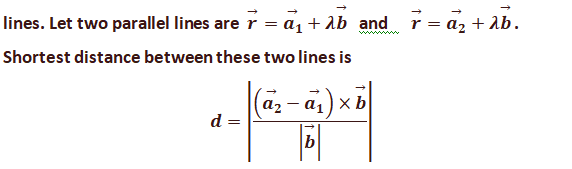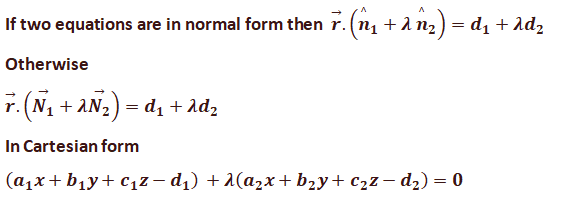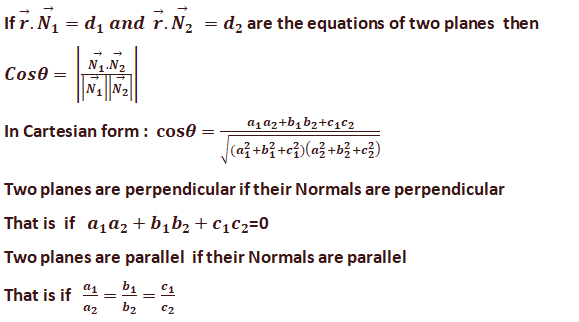### Resource Centre Mathematics

Resource Centre Mathematics Mathematics worksheet, mathematics basic points and formulas, mathematics lesson plan, mathematics multiple choice questions Workplace Dashboard CBSE Syllabus For Session 2023-24 For  :   Classes IX & X    |   Classes XI & XII Watch Videos on Maths Solutions CLASS IX MATHEMATICS FORMULAS &  BASIC CONCEPTS

### Lesson Plan Math Class 12 Ch-11 | Three Dimensional Geometry

E- LESSON PLAN   SUBJECT MATHEMATICS    CLASS 10+2
Lesson Plan, Class XII Subject Mathematics, chapter 11, Three Dimensional Geometry , for Mathematics Teacher. Effective way of Teaching Mathematics. Top planning by the teacher for effective teaching in the class. E lesson planning for mathematics.
 Board – CBSE CLASS –XII SUBJECT- MATHEMATICS CHAPTER 11  :- Three Dimensional Geometry

TOPIC:- CHAPTER 11  :  Three Dimensional Geometry

DURATION:-

This chapter is divided into 18 modules and is completed in 20 class meetings.

PRE- REQUISITE KNOWLEDGE:-

Knowledge of simple concepts of trigonometry , geometry and algebra.
Knowledge of coordinate geometry class X.

TEACHING AIDS:-

Green Board, Chalk,  Duster, Charts, smart board, projector, laptop etc.

METHODOLOGY:-   Lecture method

OBJECTIVES:-

• Direction ratios and direction cosines of a line joining two points.
• Cartesian and vector equation of a line.
• Coplanar and skew lines.
• Angle between two lines. Conditions at which two lines are parallel and perpendicular.
• Shortest distance between two lines. Shortest distance between two parallel lines.
• Cartesian and vector equation of a plane.
• Angle between two planes. Conditions at which two planes are parallel and perpendicular.
• Angle between a line and a plane.
• Distance of a point from a plane.

PROCEDURE :-

Start the session by asking the questions related to the scalar and vector quantities, their scalar product, vector product and their scalar triple product. Now introduce the topic Three dimensional geometry  step by step as follows.
 S. No. 1 Define direction angles, cosines and direction ratios. Explain the concept  by taking an example of a general vector. Direction angles: These are the angles made by the vector with the positive direction of the axis. Direction cosines: cosines of the direction angles are called direction cosines. Direction ratios: The terms which are proportional to the direction cosines are called direction ratios.2 Equation of a line in space. Case I : Equation of a line passing through the point A(x1, y1, z1) and3 Case II : Equation of a line passing through the two points A(x1, y1, z1) and B(x2, y2, z2) is given by4 Angle between two lines In Vector form let equations are56 If two lines in space intersect each other then shortest distance between them =0, If two lines in one plane are parallel then shortest distance is the perpendicular distance between them. Skew lines:- In space there are lines which are neither parallel nor intersecting such lines are non-coplanar and are called skew lines. 78 Coplanar lines:- When two lines are parallel then they are called coplanar9 Plane:- A plane is determined uniquely if any one of the following is known. a) When normal to the plane and its distance from the origin is given. That is equation of the plane in Normal form. b) When it passes through a point and is perpendicular to the given direction. c) It passes through the three non- collinear points. 1011 Equation of the plane perpendicular to the given vector and passing through a given point.12 Equation of the plane passing through the three non- collinear points.13 Intercept form of equation of line:Where a, b, c are the intercepts made by the plane on the x-axis, y-axis, z-axis respectively. 14 Equation of the plane passing through the intersection of two planes.15 Coplanarity of two lines16 Angle between two planes17 Distance of a point A(x1, y1, z1) from the plane ax + by + cz = d is18 Angle between a line and a planeEXPECTED OUTCOMES:-

After studying this lesson students should know the
• direction cosines and direction ratios of a line joining two points.
• equation of line passing through one point and two points.
• equation of plane passing through one point and three points
• Students should knows the angle between two lines between two planes between a line and a plane.
• shortest distance between two lines
• shortest distance between two parallel lines.
• perpendicular distance of the point from the plane.
STUDENTS DELIVERABLES:-

Review questions given by the teacher. Students should prepare the presentation on different equations of line and plane in vector and Cartesian form. Solve NCERT problems with examples.

EXTENDED LEARNING:-

Students can extend their learning in Mathematics through the RESOURCE CENTRE
Students can also find many interesting topics on mathematics at cbsemathematics.com

ASSESSMENT TECHNIQUES:-
• Assignment sheet will be given as home work at the end of the topic.
• Separate sheets which will include questions of logical thinking and Higher order thinking skills will be given to the above average students.
• Class Test , Oral Test , worksheet and  can be made the part of assessment.
• Re-test(s) will be conducted on the basis of the performance of the students in the test.

🙏

1.